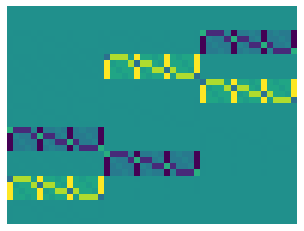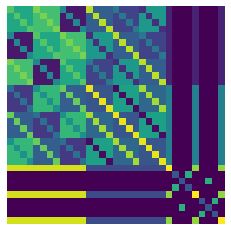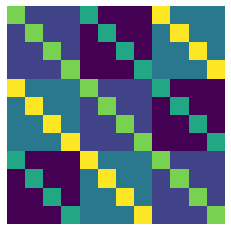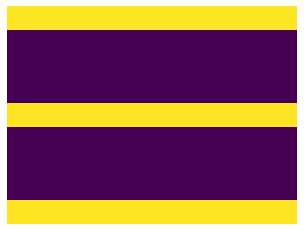# Combining Representations from Different Groups (experimental)¶

## Direct Product groups¶

It is possible to combine representations from different groups. These kinds of representations are relevant when there are multiple structures in the data. For example, a point cloud is a set of vectors which transforms both under permutations and rotations of the vectors. We can formalize this as $$V_{S_n}\otimes V_{SO(3)}$$ or in tensor notation $$T_1^{S_n}\otimes T_1^{SO(3)}$$. While this object could be expressed as the representation of the product group $$V_{S_n \times SO(3)}$$, other objects like $$T_k^{S_n}\otimes T_j^{SO(3)}$$ can not be so easily.

Nevertheless, we can calculate the symmetric bases for these objects. For example, maps from vector edge features $$T_1^{SO(3)}\otimes T_2^{S_n}$$ to matrix node features $$T_2^{SO(3)}\otimes T_1^{S_n}$$ can be computed:

:

from emlp.groups import *
from emlp.reps import T,vis,V,Scalar

repin,repout = T(1)(SO(3))*T(2)(S(4)),T(2)(SO(3))*T(1)(S(4))
print(repin,"-->", repout)

V_SO(3)⊗V²_S(4) --> V²_SO(3)⊗V_S(4)

:

vis(repin,repout,cluster=False)Or perhaps you would like equivariant maps from two sequence of sets and a matrix (under 3D rotations) to itself. You can go wild.

:

rep = 2*T(1)(Z(3))*T(1)(S(4))+T(2)(SO(3))
print(f"Rep: {rep}")
print(f"Linear maps: {rep>>rep}")

Rep: V²+2V_S(4)⊗V_Z(3)
Linear maps: V⁴+4V_S(4)⊗V_Z(3)⊗V²_SO(3)+4V²_S(4)⊗V²_Z(3)

:

vis(rep,rep)The kronecker product of the individual solutions for different groups can be seen in these basis matrices, in the top left hand corner we have a circulant matrix of deep set solutions (identity + background)

:

vis(V(Z(3))*V(S(4)),V(Z(3))*V(S(4)))And in the bottom left we have maps $$V_{Z_3}\otimes V_{S_4} \rightarrow V_{SO(2)}^{\otimes 2}$$ of which the solutions are the product of $$V_{Z_3}\otimes V_{S_4} \rightarrow \mathbb{R}$$ (the vector $$\mathbf{1}$$) and $$\mathbb{R} \rightarrow V_{SO(2)}^{\otimes 2}$$ (the flattened $$I_{3\times 3}$$)

:

vis(V(Z(3))*V(S(4)),T(2)(SO(3)),False)## Wreath Products (coming soon)¶

These are all examples of tensor products of representations, which are in turn a kind of representation on the direct product group $$G=G_1\times G_2$$. There are other ways of combining groups however, and for many hierarchical structures there is a larger group of symmetries $$G_1\wr G_2$$ that contains $$G_1 \times G_2$$ but also other elements. These so called wreath products in the very nice paper Equivariant Maps for Hierarchical Structures. Support for the wreath product of representations is not yet implemented but if this is something that would be useful to you, send me an email.